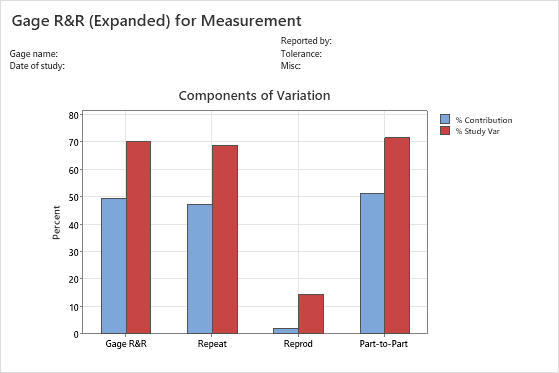# Interpret the key results for Expanded Gage R&R Study

Complete the following steps to interpret an expanded gage R&R study. Key output includes variability estimates, and graphs of the measurements and measurement variability.

## Step 1: Use the ANOVA table to identify significant factors and interactions

Use the ANOVA table to identify which sources of variability are significant. The ANOVA table includes the following terms in the Source column:
• Part: The variation that is from the parts.
• Operator: The variation that is from the operators.
• Nested factors: The variation that is from nested factors. Two factors are nested when each level of one factor occurs with only one level of the other factor. For example, if two operators measure two different, but similar, sets of parts, parts are nested under operator, and is indicated by Part (Operator). In this case, if the average measurement of different parts by each operator varies greatly, then Part (Operator) will be a significant source of variability.
• Interaction terms, such as Part*Operator: The variation that is from an interaction. For example, an interaction exists when an operator measures different parts differently.
• Error or repeatability: The variation that is not explained by part, operator, or other terms in the model.

If the p-value for an interaction is 0.05 or higher, Minitab omits the interaction because it is not significant and generates a second ANOVA table without the insignificant interactions.

## Step 2: Assess the variation for each source of measurement error

Use the variance components (VarComp) and %Contribution to assess the variation for each source of measurement error. The sources are as follows:
• Total Gage R&R: The sum of the repeatability and the reproducibility variance components.
• Repeatability: The variability in measurements when the same operator measures the same part multiple times.
• Reproducibility: The variability in measurements when different operators measure the same part at the various conditions defined by the other factors in the model. The Reproducibility term can be divided further into Operator, Operator*Part, and other main effects and interaction effects.
• Part-to-Part: The variability in measurements due to different parts. In addition to Part, other factors might be used to calculate part-to-part variation.

Ideally, very little of the variability should be due to repeatability and reproducibility. Differences between parts (Part-to-Part) should account for most of the variability.Key Result: Components of Variation graph The components of variation graph shows the variation from the sources of measurement error. Minitab displays bars for %Tolerance when you enter a tolerance value, and Minitab displays bars for %Process when you enter a historical standard deviation. This graph shows that part-to-part variability is approximately the same as the variability from repeatability and reproducibility. The total gage R&R variation is much higher than 30% and is unacceptable.

## Step 3: Examine the graphs for more information on the gage study

The gage R&R graphs provide information about the measurement system.
Components of variation graph
Shows whether the largest of component of variation is part-to-part variation.
In an acceptable measurement system, the largest component of variation is part-to-part variation.
R chart by operator
Shows whether any points fall above the upper control limit.
If the operators measure consistently, the points will fall within the control limits.
Xbar chart by operator
Shows whether most points fall beyond the control limits.
The parts that you choose for a gage R&R study should represent the typical part-to-part variability. Thus, you should expect more variation between part averages, and the graph should show that most points fall beyond the control limits.
Measurements by a single factor
Shows all measurements, arranged by one factor. This graph helps you visualize the differences between factor levels.
In addition to displaying measurements by part or by operator, an expanded gage R&R study also allows you to display graphs of measurements arranged by other factors.
Measurements by part graph
Shows whether multiple measurements for each part are close together.
Multiple measurements for each part that are close together indicate small variation between the measurements of the same part.
Measurements by operator graph
Shows whether differences between operators are small compared to the differences between parts.
A straight horizontal line across operators indicates that the mean measurements for each operator are similar. Ideally, the measurements for each operator vary an equal amount.
Interaction plots
Shows the interaction between two factors. An interaction occurs when the effect of one factor is dependent upon a second factor. This plot is the graphical analog of the F-test for an interaction term in the ANOVA table.
In addition to displaying the interaction of operator by part, an expanded gage R&R study also allows you to display graphs of other interactions.
The operator*part interaction graph
Shows whether the lines that connect the measurements from each operator are similar or whether the lines cross each other.
Lines that are coincident indicate that the operators measure similarly. Lines that are not parallel or that cross indicate that an operator's ability to measure a part consistently depends on which part is being measured. A line that is consistently higher or lower than the others indicates that an operator adds bias to the measurement by consistently measuring high or low.
By using this site you agree to the use of cookies for analytics and personalized content.  Read our policy